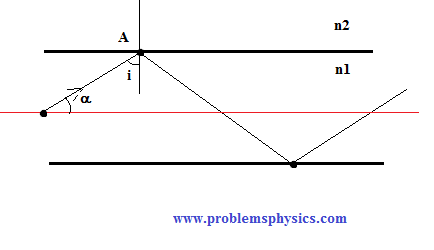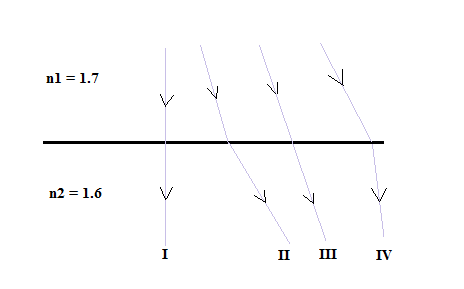# Total Internal Reflection of Light Rays at an Interface, Examples and Solutions

#### Total Internal Reflection

Let a ray of light being incident through a medium of refractive index n1 = 1.1 onto a medium of refractive index n2 = 1.0. Let i be the angle of incidence and t being the angle of refraction .According to Snell's law we can write
n1 sin(i) = n2 sin(t)
Solve for t to obtain
t = arcsin [ (n1 / n2) sin(i) ]

Let us make a table of values including different angles of incidence and calculate the angles of refraction

 i sin(t) = (n1/n2) sin(i) t = arcsin [ (n1 / n2) sin(i) ] 40 � 0.70 45.0 � 65.39 � 1.0 90.0 � 75 � 1.06 the sine of an angle cannot be greater than 1 t does not exist no refraction therefore the ray is reflected

#### Critical Angle

Because n1 is greater than n2, there is a certain angle i for which the angle of refraction is 90 �. From the table this special incidence angle, called critical angle, is equal to 65.39 �. Any ray incident at an angle greater than the critical angle will be totally reflected.Let us find a formula for this critical angle of incidence ic.
So when t = 90 � using Snell's law we write: n1 sin(ic) = n2 sin(t) = n2 sin(90�) = n2
which gives
sin(ic) = n2/n1
The angle of incidence ic = arcsin(n2/n1) is called the critical angle. For any angle of incidence greater than ic, there is no refraction; the ray is totally reflected into the medium of incidence with refractive index n1.

Example 1

A ray of light incident in water strikes the surface (assumed flat) separating water from air making an angle of 10 � with the normal to the surface. (refractive index of air = 1 and refractive index of water = 1.3)a) What is the angle of refraction?
b) What should be the angle of incidence if we want an angle of refraction not greater than 45 � ?
c) What is the critical angle?

 Solution a) Use Snell's law to write n1 sin(i) = n2 sin(t) 1.3 � sin(i) = 1 � sin(t) sin (t) = 1.3 � sin(10�) t = arcsin(1.3 � sin(10�)) ≈ 13.0 � b) t < 45 � sin t < √ 2 / 2 From Snell's law : sin t = 1.3 � sin(i) Hence : 1.3 � sin(i) < √ 2 / 2 sin i < √ 2 / 2.6 i < arcsin ( √ 2 / 2.6) i < 33.0� c) critical angle ic = arcsin (1/1.3) = 59 �

Example 2

An optical fiber is made up of a core, where light travels, made of glass of refractive index n1 = 1.5 surrounded by another layer of glass of lower refractive index n2.a) Find the refractive index n2 of the cladding so that the critical angle at the interface core cladding is 80 �.
b) α is the angle made by the ray with the axis of the fiber. For what values of α is the incident angle i larger that the critical angle found in part a) above.
 Solution a) Ic being the critical angle is given by sin(ic) = n2/n1 sin (80 �) = n2/1.5 n2 = 1.5 � sin (80 �) ≈ 1.48 b) i + α = 90 � i = 90 - α i > ic Substitute i by 90 - α in the above inequality to obtain 90 - α > ic α < 90 - ic α < 90 - 80 α < 10� For α < 10� where α is the angle that the light rays make with the axis of the optical fiber, the angle of incidence is larger that the critical angle and total international reflection takes place at the interface core-cladding.

Example 3
Which of the following is a possible path followed by a ray of light incident from n1 into n2?A) I only
B) II only
C) I and II only
D) I, II and IV only
E) IV only

 Solution Light rays are incident in medium with a refractive index 1.7 higher than the refractive index of the medium where the ray will be refracted. If the angle of incidence is equal to 0 case I, the ray is transmitted into the second medium without any change of direction. So case I is possible. If the angle of incidence is greater than 0, with n1 > n2, the angle of incidence is smaller than the angle of refraction and so II is also possible. Answer C.